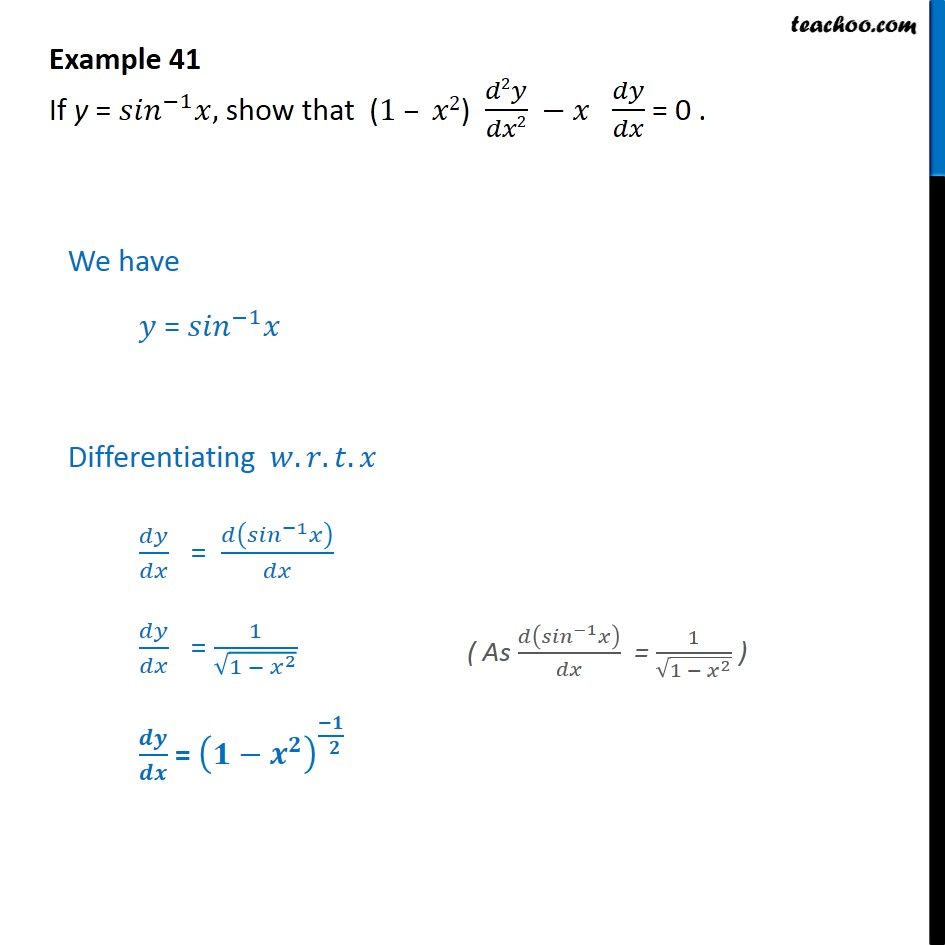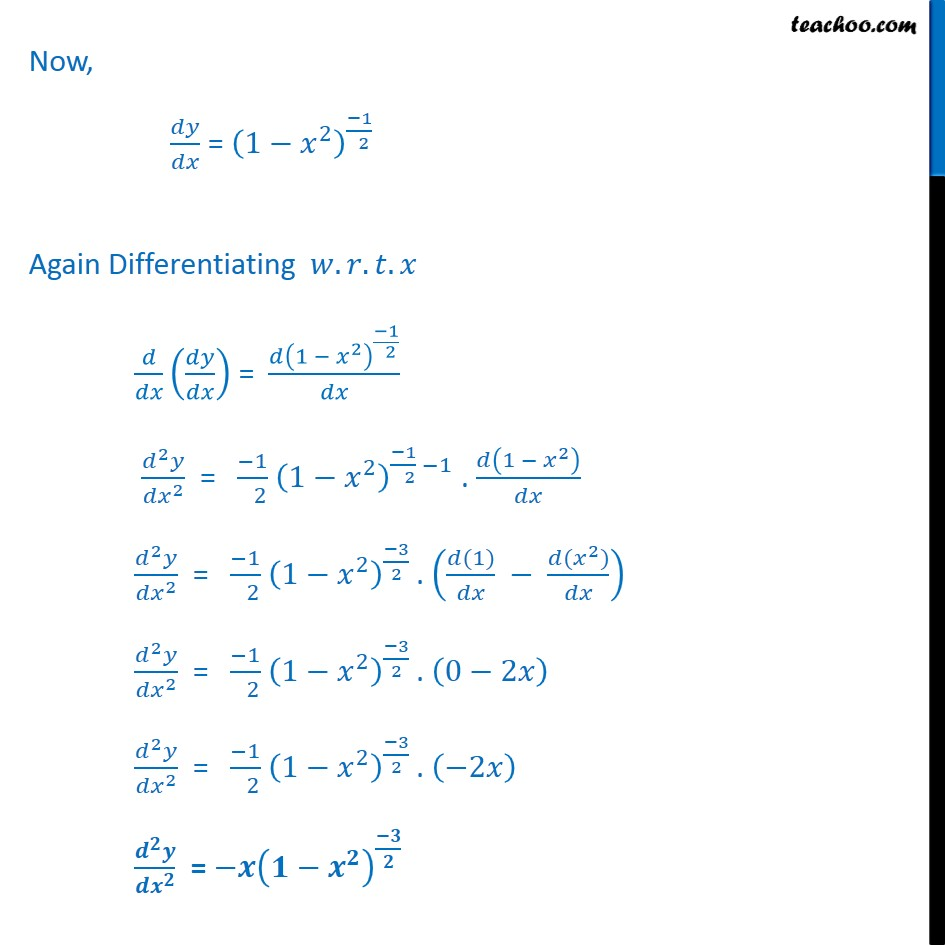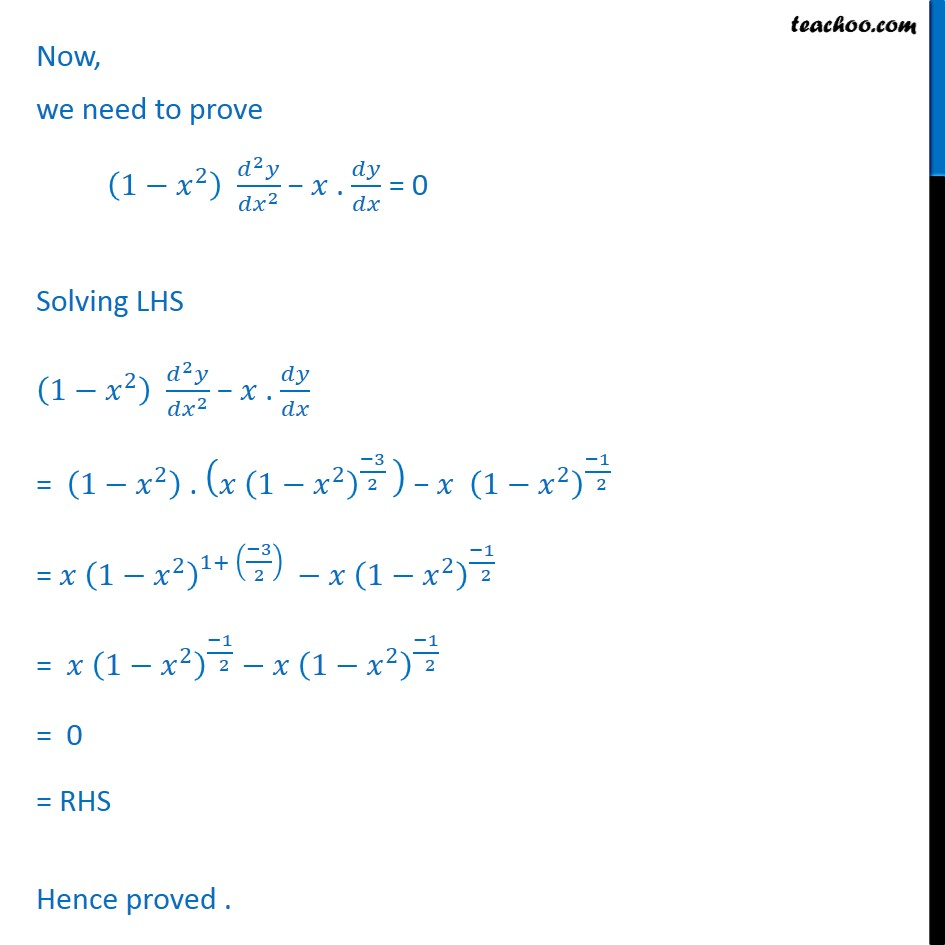1. Class 12
2. Important Question for exams Class 12
3. Chapter 5 Class 12 Continuity and Differentiability

Transcript

Example 41 If y = 1 , show that (1 2) 2 2 = 0 . We have = 1 Differentiating . . . = 1 = 1 1 2 = Now, = 1 2 1 2 Again Differentiating . . . = 1 2 1 2 2 2 = 1 2 1 2 1 2 1 . 1 2 2 2 = 1 2 1 2 3 2 . (1) ( 2 ) 2 2 = 1 2 1 2 3 2 . 0 2 2 2 = 1 2 1 2 3 2 . 2 = Now, we need to prove 1 2 2 2 . = 0 Solving LHS 1 2 2 2 . = 1 2 . 1 2 3 2 1 2 1 2 = 1 2 1+ 3 2 1 2 1 2 = 1 2 1 2 1 2 1 2 = 0 = RHS Hence proved .

Chapter 5 Class 12 Continuity and Differentiability

Class 12
Important Question for exams Class 12